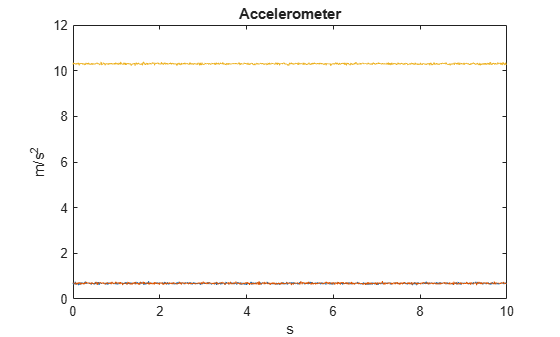# accelparams

Accelerometer sensor parameters

## Description

The `accelparams` class creates an accelerometer sensor parameters object. You can use this object to model an accelerometer when simulating an IMU with `imuSensor`. See the Algorithms section of `imuSensor` for details of `accelparams` modeling.

## Creation

### Syntax

``params = accelparams``
``params = accelparams(Name,Value)``

### Description

````params = accelparams` returns an ideal accelerometer sensor parameters object with default values.```

example

````params = accelparams(Name,Value)` configures an accelerometer sensor parameters object properties using one or more `Name-Value` pair arguments. `Name` is a property name and `Value` is the corresponding value. `Name` must appear inside single quotes (`''`). You can specify several name-value pair arguments in any order as (`Name1,Value1,...,NameN,ValueN`). Any unspecified properties take default values.```

## Properties

expand all

Maximum sensor reading in m/s2, specified as a real positive scalar.

Data Types: `single` | `double`

Resolution of sensor measurements in (m/s2)/LSB, specified as a real nonnegative scalar. Here, LSB is the acronym for least significant bit. Resolution is often referred to as Scale Factor for accelerometer.

Data Types: `single` | `double`

Constant sensor offset bias in m/s2, specified as a real scalar or 3-element row vector. Any scalar input is converted into a real 3-element row vector where each element has the input scalar value.

Data Types: `single` | `double`

Sensor axes skew in percentage, specified as a scalar, a 3-element row vector, or a 3-by-3 matrix. The diagonal elements of the matrix account for the misalignment effects for each axes. The off-diagonal elements account for the cross-axes misalignment effects. The measured state vmeasure is obtained from the true state vtrue via the misalignment matrix as:

`${v}_{measure}=\frac{1}{100}M\text{ }{v}_{true}=\frac{1}{100}\left[\begin{array}{ccc}{m}_{11}& {m}_{12}& {m}_{13}\\ {m}_{21}& {m}_{22}& {m}_{23}\\ {m}_{31}& {m}_{32}& {m}_{33}\end{array}\right]{v}_{true}$`

• If you specify the property as a scalar, then all the off-diagonal elements of the matrix take the value of the specified scalar and all the diagonal elements are 100.

• If you specify the property as a vector [a b c], then m21 = m31 = a, m12 = m32 = b, and m13 = m23 = c. All the diagonal elements are 100.

Data Types: `single` | `double`

Power spectral density of sensor noise in (m/s2/√Hz), specified as a real scalar or 3-element row vector. This property corresponds to the velocity random walk (VRW). Any scalar input is converted into a real 3-element row vector where each element has the input scalar value.

Data Types: `single` | `double`

Filter coefficients for bias instability noise generation, specified as a structure. The structure has these fields:

• `Numerator` — Numerator coefficients, specified as a real-valued vector.

• `Denominator` — Denominator coefficients, specified as a real-valued vector.

To specify coefficients for fractal noise, use the `fractalcoef` function.

Example: `struct(Numerator=1,Denominator=[1 -0.5])`

Data Types: `struct`

Instability of the bias offset in m/s2, specified as a real scalar or 3-element row vector. Any scalar input is converted into a real 3-element row vector where each element has the input scalar value.

Data Types: `single` | `double`

Integrated white noise of sensor in (m/s2)*(√Hz), specified as a real scalar or 3-element row vector. Any scalar input is converted into a real 3-element row vector where each element has the input scalar value.

Data Types: `single` | `double`

Type of random noise, specified as:

• `"double-sided"` — Random noise coefficients have a scale factor of 2.

• `"single-sided"` — Random noise coefficients have a scale factor of 1.

Data Types: `char` | `string`

Sensor bias from temperature in (m/s2)/℃, specified as a real scalar or 3-element row vector. Any scalar input is converted into a real 3-element row vector where each element has the input scalar value.

Data Types: `single` | `double`

Scale factor error from temperature in %/℃, specified as a real scalar or real 3-element row vector with values ranging from 0 to 100. Any scalar input is converted into a real 3-element row vector where each element has the input scalar value.

Data Types: `single` | `double`

## Examples

collapse all

Generate accelerometer data for an imuSensor object from stationary inputs.

Generate an accelerometer parameter object with a maximum sensor reading of 19.6 $\mathrm{m}/{\mathrm{s}}^{2}$ and a resolution of 0.598 $\left(\mathrm{mm}/{\mathrm{s}}^{2}\right)/\mathrm{LSB}$. The constant offset bias is 0.49 $m/{s}^{2}$. The sensor has a power spectral density of 3920 $\left(\mu \mathrm{m}/{\mathrm{s}}^{2}\right)/\sqrt{\mathrm{Hz}}$. The bias from temperature is 0.294 $\left(\mathrm{m}/{\mathrm{s}}^{2}\right)$$/{}^{0}C$. The scale factor error from temperature is 0.02%$/{}^{0}C$. The sensor axes are skewed by 2%.

`params = accelparams('MeasurementRange',19.6,'Resolution',0.598e-3,'ConstantBias',0.49,'NoiseDensity',3920e-6,'TemperatureBias',0.294,'TemperatureScaleFactor',0.02,'AxesMisalignment',2);`

Use a sample rate of 100 Hz spaced out over 1000 samples. Create the imuSensor object using the accelerometer parameter object.

```Fs = 100; numSamples = 1000; t = 0:1/Fs:(numSamples-1)/Fs; imu = imuSensor('SampleRate', Fs, 'Accelerometer', params);```

Generate accelerometer data from the imuSensor object.

```orient = quaternion.ones(numSamples, 1); acc = zeros(numSamples, 3); angvel = zeros(numSamples, 3); accelData = imu(acc, angvel, orient);```

Plot the resultant accelerometer data.

```plot(t, accelData) title('Accelerometer') xlabel('s') ylabel('m/s^2')```## Version History

Introduced in R2018b